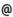## "Babes-Bolyai" University of Cluj-Napoca Faculty of Mathematics and Computer Science

 Mathematical logic, set theory and arithmetics
 Code Semes-ter Hours: C+S+L Credits Type Section MML0003 1 2+2+0 6 compulsory Matematică MML0003 1 2+2+0 6 compulsory Matematică informatică MML0003 1 2+2+0 6 compulsory Matematici aplicate
 Teaching Staff in Charge
 Prof. MARCUS Andrei, Ph.D., marcusmath.ubbcluj.ro
 Assoc.Prof. COVACI Rodica, Ph.D., rcovacimath.ubbcluj.ro
 Lect. SACAREA Cristian, Ph.D., csacareamath.ubbcluj.ro
 Aims An introduction to the elements of mathematical logic concerning the logic of sentences and predicates as a completion of the high school knowledge. Presentation of Cantor's theory of sets. Study of binary relations and functions. Considerations on cardinal numbers, finite and countable sets and ordinal numbers.
 Content 1. Elements of mathematical logic: propositional calculus, logical operators and formulae, identic true and identic false formulae, logical implication and logical equivalence, decision problem; predicate calculus, quantifiers, theorems. 2. Sets, relations, functions: set algebra, binary relations, equivalence relations and partitions, functions, one-to-one correspondence, the kernel of a function, factorization theorems, ordered sets, lattices, homomorphisms, direct product and exponentiation of sets and functions. 3. Cardinal numbers: definition, operations with cardinal numbers, ordering of cardinal numbers, countable and noncountable sets, infinite and finite sets, the set of natural numbers (the Frege-Russell construction and Peano's axiomatic study).
 References 1. M. BECHEANU s.a.: Algebra pentru perfectionarea profesorilor, Ed. Didactica si Pedagogica, Bucuresti 1983. 2. I.T. ADAMSON: A Set Theory Workbook, Birkhauser, Boston, 1998. 3. S. BILANIUK: A Problem Course in Mathematical Logic, Trent University, Ontario 2003 4. G. GRATZER: General Lattice Theory, Birkhauser, Boston 1998. 5. P.R. HALMOS: Naive Set Theory, D. Van Nostrand Company Inc. Princeton 1967. 6. C. NASTASESCU: Introducere in teoria multimilor, Ed. Didactica si Pedagogica, Bucuresti 1981. 7. Y.I. MANIN: A Course in Mathematical Logic, Springer-Verlag, New York 1977 8. S.G. KRANTZ: Logic and Proof Techniques for Computer Science, Birkhauser Boston 2002. 9. I.A. LAVROV, L.L. MAKSIMOVA: Probleme de teoria multimilor si logica matematica, Ed. Tehnica, Bucuresti 1974. 10. A. MARCUS, C. SZANTO, L. TOTH: Logika es halmazelmelet, Sapientia Kiado, Kolozsvar 2004. 11. A. HAJNAL, L. CSIRMAZ: Matematikai logika, Egyetemi jegyzet, ELTE, Budapest 1994. 12. A. HAJNAL, P. HAMBURGER: Halmazelmelet, Tankonyvkiado, Budapest 1994. 13. P. KOMJATH: Halmazelmelet, Egyetemi jegyzet, ELTE Budapest 1999. 14. P. KOMJATH: Matematikai logika, Egyetemi jegyzet, ELTE Budapest 2000.
 Assessment Two control papers throughout the semester (each representing 20% of the final mark) and oral exam at the end of the semester (representing 60% of the final mark).